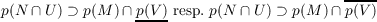#### Vol. 73, No. 1, 1977

 Download this articleFor screen For printingRecent Issues Vol. 325: 1 Vol. 324: 1  2 Vol. 323: 1  2 Vol. 322: 1  2 Vol. 321: 1  2 Vol. 320: 1  2 Vol. 319: 1  2 Vol. 318: 1  2Online Archive Volume: Issue:The Journal Subscriptions Editorial Board Officers Contacts Submission Guidelines Submission Form Policies for Authors ISSN: 1945-5844 (e-only) ISSN: 0030-8730 (print) Special Issues Author Index To Appear Other MSP Journals
A general Rudin-Carlson theorem in Banach-spaces

### Walter Roth

Vol. 73 (1977), No. 1, 197–213
##### Abstract

Let K be a closed subspace in a real or complex normed linear space L. The “Main Interpolation Problem” as formulated by L. Asimow reads as follows: Given a bounded convex neighborhood V of 0 in L and a bounded closed convex U containing 0, their polars V 0 and U0 in the dual Lof L, define the functionals on LpV K(x) = sup(x,V 0 K0) and pU(x) = sup(x,U0). For x0 L we are looking for an element x L satisfying

1. x x0 K(x|K0 = x0|K0) and
2. pU(x) = pV K(x0) (exact solution), respectively
3. pU(x) pV K(x0) + 𝜖 for given 𝜖 > 0 (approximate solution).

The problem is formulated in a different but equivalent way in this paper using the canonical projection p from L to L∕K. For a real linear subspace M of L, a convex cone N in M and bounded closed convex neighborhoods U and V we prove conditions in terms of the dual space of L which are necessary and sufficient for the inclusions({} means the topological interior, {}, the closure).

Primary: 46B99
##### Milestones